# How to use an Infrared Proximity Sensor (Extra-Long-Range)

The sharp infrared distance proximity sensor is a sensor for distance measurements. This sensor is typically used for measuring larger ranges(approximately 100 – 500 cm(39″ to 197″)), although it is quite accurate.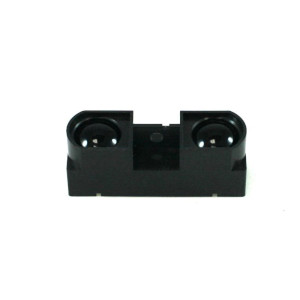## How it works​

The component uses a specific light sensor to detect a light wavelength in the Infrared (IR) wave spectrum. The intensity of the light is received when an object is close to the sensor. The light bounces off the object and into the light sensor, which results in a change to the intensity.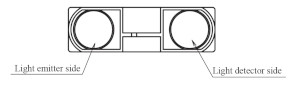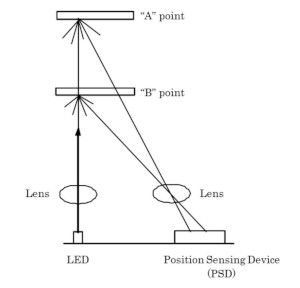## Wiring​

• Red Wire: connected to 5V
• Blue Wire: connected to 5V
• Black Wire: connected to ground
• Yellow Wire: connect to ground
• Green Wire: connect to an analog pin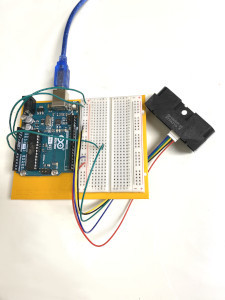## Wiring Guide​

• Connect the Voltage and Ground Wires to the breadboard
• Connect the component wire (green wire) to an analog pin (ex: A0)
• Under the Tools folder select you board and pick the port you are using to upload the code onto the Arduino
• Watch the change in data from the Serial Monitor found under the Tools tab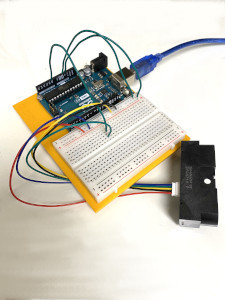## Programming​

The following code demonstrates how the short proximity sensor works and measures the distance when the sensor is moved towards or away from an object, within the range of 100-500cm. If you want to skip the steps, you can jump to the Full Code.

Step 1: Create variables for the sensors pin and a variable to store the data that is collected

``int sensor = A0; //sensor pin connection``

Step 2: Set up serial communication, set the sensor pin as an INPUT.

``void setup() { Serial.begin(9600); // start the serial port pinMode(sensor, INPUT); //setting the pin to the Arduino}``

Step 3: Read the data from the sensor, and convert the data into a volts measurement

``void loop() { //5V float volts = analogRead(sensor)*0.0048828125;  // value from sensor * (5/1024)``

Step 4: Now convert the volts measurement into distance, a measurement we understand. This calculation is found in the datasheet gragh of the component and can be found here!

``int distance = 13*pow(volts, -1); // worked out from datasheet graph delay(1000); // slow down serial port ``

Step 5: Finally use the distance we calculated and display ONLY when the distance is less than or equal to its max limit.

``if (distance >= 100 && distance <= 500){   Serial.println(distance);   // prints the distance }}``

## Full Code​

``int sensor = A0; //sensor pin connectionvoid setup() { Serial.begin(9600); //start the serial port pinMode(sensor, INPUT); //setting the pin to the Arduino}void loop() { //5V float volts = analogRead(sensor)*0.0048828125;  // value from sensor * (5/1024) int distance = 13*pow(volts, -1); // worked out from datasheet graph delay(1000); // slow down serial port   if (distance >= 100 && distance <= 500){   Serial.println(distance);   // prints the distance }}``

## Output​

Serial Monitor will display distance that is measure from the proximity sensor, only if the distance measure is greater than or equal to 100 and less than or equal to the max limit which is 500cm for this exact component.